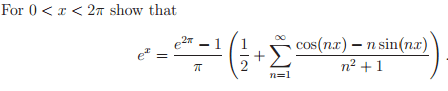# Fourier series (maybe) of e^x from 0 to 2pi

Hey,
I have to show:Should I try to find the fourier series from -2pi to 2pi? I have tried this already but I can't seem to get rid of the cos(nx/2) and sin(nx/2) to turn them into just sin(nx) and cos(nx) and the denominator stays as (n^2+4 instead of n^2+1.

Any suggestions would be very helpful.

## Answers and Replies

It's probably easiest to use the complex Fourier series.
$$f(x)=e^x=\sum_{-\infty}^\infty C_n e^{in\pi x/\ell}\text{, where}\\ C_n=\frac{1}{2\ell}\int_{-\ell}^\ell f(x)e^{-in\pi x/\ell}\, \mathrm{d}x\text{.}$$
The range of integration will be a little different in your case. You can picture ##e^x## as being a function that repeats every ##2\pi##. Effectively, ##\ell=\pi##, but you're not integrating symmetrically about the origin.
$$C_n=\frac{1}{2\pi}\int_{0}^{2\pi} e^xe^{-in\pi x/\pi}\, \mathrm{d}x=\frac{1}{2\pi}\int_{0}^{2\pi}e^{(1-in)x}\, \mathrm{d}x\\ =\frac{1}{2\pi(1-in)}e^{(1-in)x}\big|_0^{2\pi}\\ =\frac{e^{2\pi}-1}{2\pi(1-in)}=C_n$$
I should probably let you handle it from here. Use the expression given for ##f(x)## above.